﻿ 医疗保险对长期贫困的影响：作用机制与动态模拟《财经研究》
2022第48卷第8期

The Impact of Health Insurance on Long-term Poverty: Mechanism and Dynamic Simulation
Zhao Guiqin, Kong Xiangzhao, Chen Ying
School of Finance, Shanghai University of Finance and Economics, Shanghai 200433, China
Summary: Under the current standards, China has eliminated absolute poverty and has achieved decisive progress and staged victory in poverty alleviation. After 2020, consolidating the achievements of poverty alleviation and reducing long-term poverty will be the focus of anti-poverty. Therefore, it is necessary to analyze the impact of different poverty reduction policies on the long-term poverty rate, depth of poverty and costs of long-term poverty reduction. This will help to optimize existing poverty reduction policies and allocate resources for poverty alleviation efficiently. This paper develops a dynamic theoretical model to compare vulnerability to poverty and investment decisions of households with different asset levels in the three cases of government cash subsidy, health insurance and health insurance with premium subsidy. On this basis, this paper further analyzes the changing trend of long-term poverty rate, depth of poverty and costs of long-term poverty reduction in the three cases. It is found that: First, health insurance has an ex-post vulnerability reduction effect, especially for families who are non-poor vulnerable. Second, for families who are poor and non-poor vulnerable, health insurance can increase productive investment through the ex-ante investment incentive effect. Third, compared with government cash subsidy, “government cash subsidy + premium subsidy” significantly lowers the long-term poverty rate, depth of poverty and costs of long-term poverty reduction. This paper contributes to the existing literature in three ways: First, this paper develops a dynamic theoretical model to compare vulnerability to poverty and investment decisions of households. Further, it analyzes the ex-ante incentive effect of health insurance on the production investment of poor and non-poor vulnerable families, enriching the theoretical research on the mechanism of health insurance in poverty reduction and prevention of returning to poverty. Second, from the perspective of long-term poverty reduction, this paper discusses the mechanism of health insurance on the poverty dynamics of different groups, especially the impact of health insurance on the inter-temporal capital dynamics of non-poor vulnerable families, and deepens the understanding of the function of health insurance to restrain the return to poverty. Third, on the basis of government cash subsidy, this paper introduces health insurance and further explores the synergistic effect of the two on long-term poverty reduction.
Key words: health insurance    vulnerability to poverty    investment incentive    long-term poverty reduction

（一）生产函数

 $f\left({k}_{t}\right)=\left\{\begin{array}{c}{f}^{H}={\alpha }^{H}{k}_{t}^{{\gamma }_{H}}+ \underline{f}^{H},{k}_{t} > \tilde{k}\\ {f}^{L}={\alpha }^{L}{k}_{t}^{{\gamma }_{L}}+{\underline{f}}^{L},{k}_{t}\leqslant \tilde{k}\end{array}\right.$ (1)

（二）基准模型

 $\begin{gathered} \mathop {max}\limits_{\left\{ {{c_t}} \right\}_{t = 0}^\infty } E\left[ {\sum\limits_{t = 0}^\infty {{\beta ^t}u\left( {{c_t}} \right)} } \right] \end{gathered}$
 $s.t.\; \left\{\begin{array}{c}{c}_{t}+{i}_{t}=f\left({k}_{t}\right)+(1-\delta ){k}_{t},\forall t=\mathrm{0,1},2,\dots \\ {k}_{t+1}=\left(1-{\varepsilon }_{t}\right){i}_{t}\end{array}\right.$ (2)

 ${V}_{N}\left({k}_{t}\right)=\underset{{c}_{t}}{max}\left\{u\left({c}_{t}\right)+\beta {E}_{t}\left[{V}_{N}\left({k}_{t+1}\right)\right]\right\}$ (3)

${V}_{N}\left({k}_{t}\right)$ 中的下标 $N$ 表示经济中不存在医疗保险。本文所采用的非凸生产函数 $f\left(\cdot \right)$ 决定了一高一低两个非零均衡和“贫困陷阱”的存在。另外， $k=0$ 显然是一个均衡，但是在本文中没有意义，文中所述的均衡全都为非零均衡。在两重均衡模型中会存在一个Micawber阈值（Zimmerman和Carter，2003），本文称之为临界资产水平，资产水平低于临界资产水平的个体必然陷入贫困，而资产水平高于临界资产水平的个体也可能因为外生疾病冲击而陷入“贫困陷阱”。为了方便说明，我们将个体的贫困脆弱性定义为：在 $T$ 期内，初始资产水平为 ${k}_{0}$ 的个体落入“贫困陷阱”的概率，即个体在任意第 $t\leqslant T$ 期的资产水平低于临界资产水平的概率，具体为：

 ${p}_{T}\left({k}_{0}\right)=\mathit{Pr}[{U}_{t=1}^{t=T}({k}_{t} < \mathrm{临}\mathrm{界}\mathrm{资}\mathrm{产}\mathrm{水}\mathrm{平})]$ (4)

 ${u}'\left({c}_{t}\right)=\beta {E}_{t}\left[{V}_{N}'\left({k}_{t+1}\right)\left(1-{\varepsilon }_{t}\right)\right]$ (5)

（三）引入医疗保险机制的家庭资产随机增长模型

 $d\left({\varepsilon }_{t}\right)=\eta \cdot \mathit{max}\left\{{\varepsilon }_{t}-s,0\right\}$ (6)

 $\underset{{\left\{{c}_{t}\right\}}_{t=0}^{\infty },{\left\{{I}_{t}\right\}}_{t=0}^{\infty }}{max}E\left[{\sum }_{t=0}^{\infty }{\beta }^{t}u\left({c}_{t}\right)\right]$
 s.t.\; \left\{ \begin{aligned} &{c}_{t}+{i}_{t}+p{I}_{t}= f\left({k}_{t}\right)+(1-\delta ){k}_{t} \\ &{k}_{t+1}=\left(1-{\varepsilon }_{t}\right){i}_{t}+d\left({\varepsilon }_{t}\right){I}_{t} \\ &{c}_{t}\geqslant 0,\;0\leqslant {I}_{t}\leqslant {k}_{t} \end{aligned} \right. (7)

 ${V}_{I}\left({k}_{t}\right)=\underset{{c}_{t},{I}_{t}}{max}\left\{u\left({c}_{t}\right)+\beta {E}_{t}\left[{V}_{I}\left({k}_{t+1}\right)\right]\right\}$ (8)

${V}_{I}\left({k}_{t}\right)$ 中下标I表示经济中引入了医疗保险机制。

 ${u}'\left({c}_{t}\right)=\beta {E}_{t}\left[{V}_{I}'\left({k}_{t+1}\right)\left(1-{\varepsilon }_{t}\right)\right]={\lambda }_{I}\left({k}_{t+1}\right)$ (9)
 $\text{}p{\lambda }_{I}\left({k}_{t+1}\right)=\beta {E}_{t}\left[{V}_{I}'\left({k}_{t+1}\right)d\left({\varepsilon }_{t}\right)\right]$ (10)

${\lambda }_{I}\left({k}_{t+1}\right)$ 表示经济中引入了医疗保险机制后资产的（期望）影子价格。式（9）表明，个体在第 $t$ 期的最优消费 ${c}_{t}^{*}$ 使得个体的边际效用等于投资的边际收益。式（10）等号左右两侧分别表示医疗保险的边际成本和边际收益，即个体在 $t$ 期的最优投保额 ${I}_{t}^{*}$ 使得保险的边际成本等于边际收益。

（四）模型求解

 函数形式 符号 参数值 参数含义 参数来源 ${f}^{H}={\alpha }^{H}{k}_{t}^{ {\gamma }_{H} }+\underline{f}^{H}$ ${\gamma }^{H}$ $0.56$ 高技术水平边际生产弹性 参考Janzen等（2021）、Barrett等（2008）、Barrett和Carter（2013） $\underline{f}^{H}$ $0.70$ 高技术水平最低产出 ${\alpha }^{H}$ $1.45$ 高技术水平生产率 ${f}^{L}={\alpha }^{L}{k}_{t}^{ {\gamma }_{L} }+\underline{f}^{L}$ ${\gamma }^{L}$ $0.28$ 低技术水平边际生产弹性 $\underline{f}^{L}$ $2.80$ 低技术水平最低产出 ${\alpha }^{L}$ $1.25$ 低技术水平生产率 $u\left({c}_{t}\right)=\dfrac{ { {c}_{t} }^{1-\sigma }-1}{1-\sigma }$ $\sigma$ $1.5$ 相对风险厌恶系数 Janzen等（2021） $\delta$ $0.1$ 资产折旧因子 Liao等（2020） $\beta$ $0.95$ 效用折现因子 Janzen等（2021） $d\left({\varepsilon }_{t}\right)=\eta \mathit{max}\left\{ {\varepsilon }_{t}-s,0\right\}$ $\eta$ $70\%$ 赔付率 政府相关文件 $s$ $5\%$ 免赔率 $p=E\left[d\left({\varepsilon }_{t}\right)\right]$ $p$ $2.43\%$ 保费费率 根据公式计算 外生疾病冲击 $\varepsilon =\left\{\mathrm{0.0,0.05,0.10,0.15,0.20,0.25,0.30,0.35,0.40,0.45,0.50,0.55,0.60}\right\}$ $\mathit{Pr}(\varepsilon )=\left\{50\%,25\%,10\%,6\%,3\%,2\%,1\%,0.5\%,0.5\%,0.5\%,0.5\%,0.5\%,0.5\%\right\}$

1. 生产函数及参数设定。本文采用形如式（1）的生产函数来描绘生产活动，参考Janzen等（2021），以上标HL分别代表高技术水平和低技术水平，边际产出弹性分别设定为 ${\gamma }^{H}=0.56$ ${\gamma }^{L}=0.28$ ，生产率分别设定为 ${\alpha }^{H}=1.45$ ${\alpha }^{L}=1.25$ ，不同技术水平对应的最低产出分别设定为 $\underline{f}^{H}=0.70$ $\underline{f}^{L}=2.80$ ，二者之差为提升技术水平所需的成本。

2. 效用函数及基础参数设定。参照Janzen等（2021），本文采用CRRA效用函数 $u\left({c}_{t}\right)=\dfrac{{{c}_{t}}^{1-\sigma }-1}{1-\sigma }$ ，相对风险规避系数设定为 $\sigma =1.5$ ，效用折扣因子设定为 $\beta =0.95$ 。参考Liao等（2020），资产折旧因子设定为 $\delta =0.1$

3. 医疗保险参数设定。参考《山西省人民政府关于进一步完善城乡居民医疗保险政策的通知》（晋政发36号）和《贵州省整合城乡居民基本医疗保险制度实施方案》（黔府办发52号）中的有关规定，设定赔付比例 $\eta =70\%$ ，免赔率 $s=0.05$ ，保险费率采用精算公平费率。

4. 外生疾病冲击的概率分布设定如表1所示。

（一）医疗保险的贫困脆弱性降低效应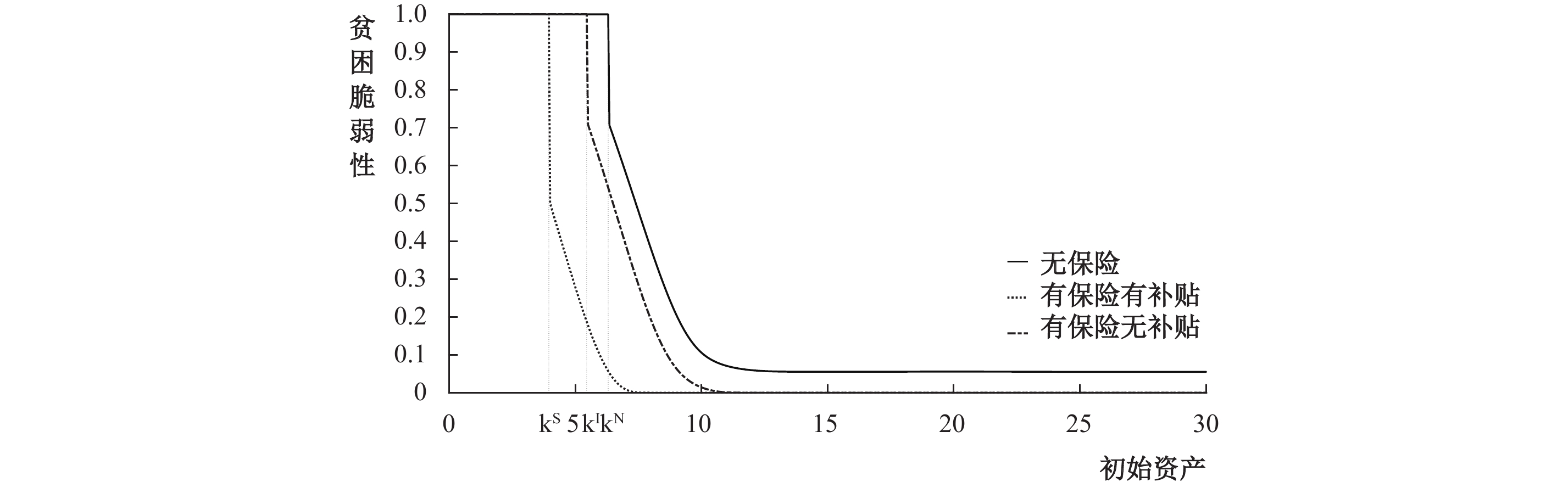图 1 不同情形下的贫困脆弱性大小

（二）医疗保险的投资激励效应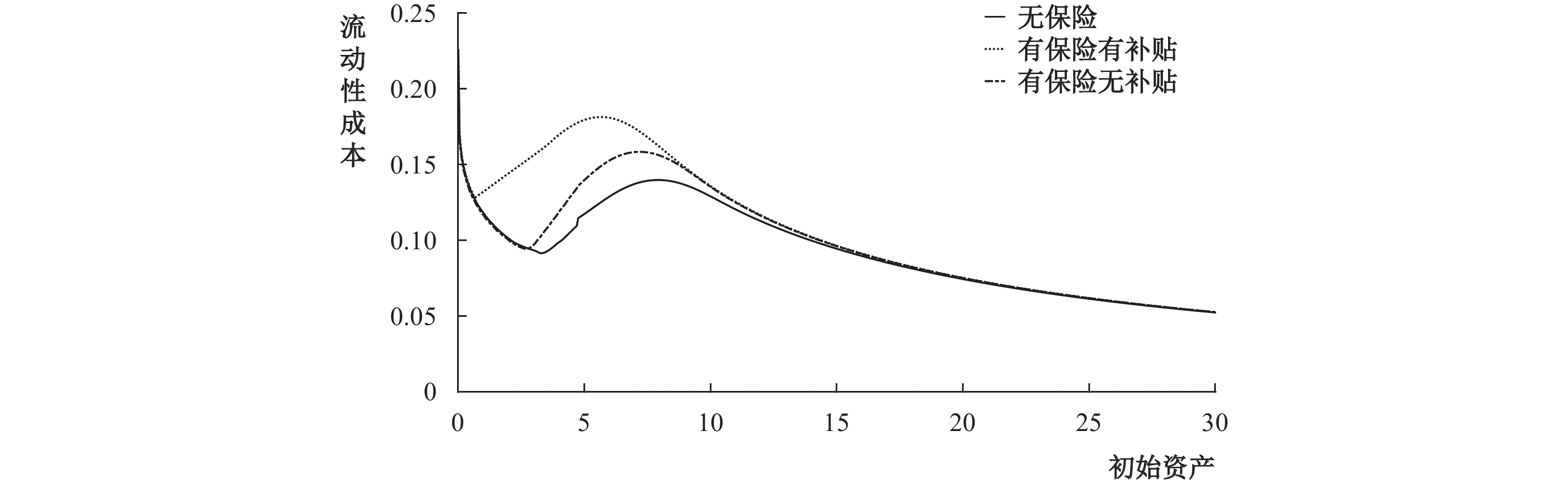图 2 不同情形下的流动性成本大小图 3 不同情形下的保险需求函数图 4 不同情形下的净投资大小

（一）经济中长期贫困的动态模拟

 变量名 观测值 均值 标准差 最小值 25%分位数 中位数 75%分位数 最大值 家庭人均年收入（单位：元） 6204 12253.27 9009.16 100 5196.67 10000 16731.67 40000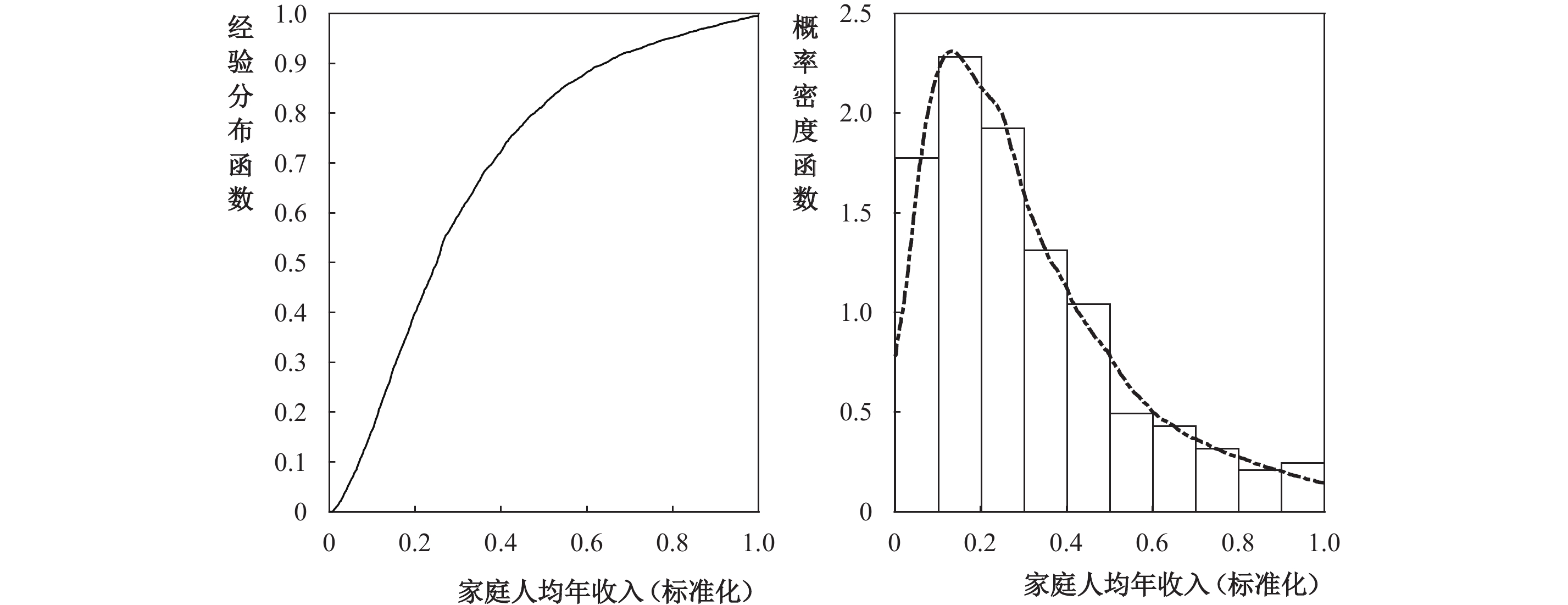图 5 农村家庭人均年收入情况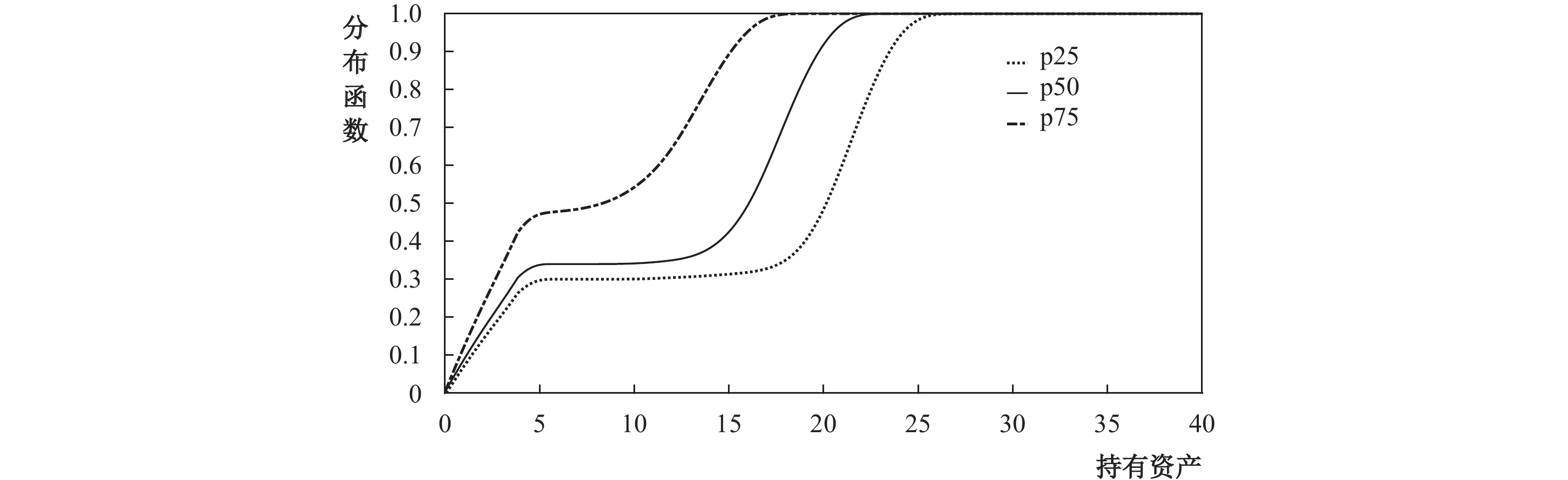图 6 模拟分布结果

（二）医疗保险对长期贫困动态的影响

 $FGT=\frac{1}{n}{\sum }_{i=1}^{n}\left(\frac{{k}^{N}-{k}_{\left(i\right)}}{{k}^{N}}\cdot {1}_{\left\{{k}_{\left(i\right)} < {k}^{N}\right\}}\right)\times 100\%$ (11)图 7 贫困家庭占比随时间变化情况图 8 贫困深度随时间变化情况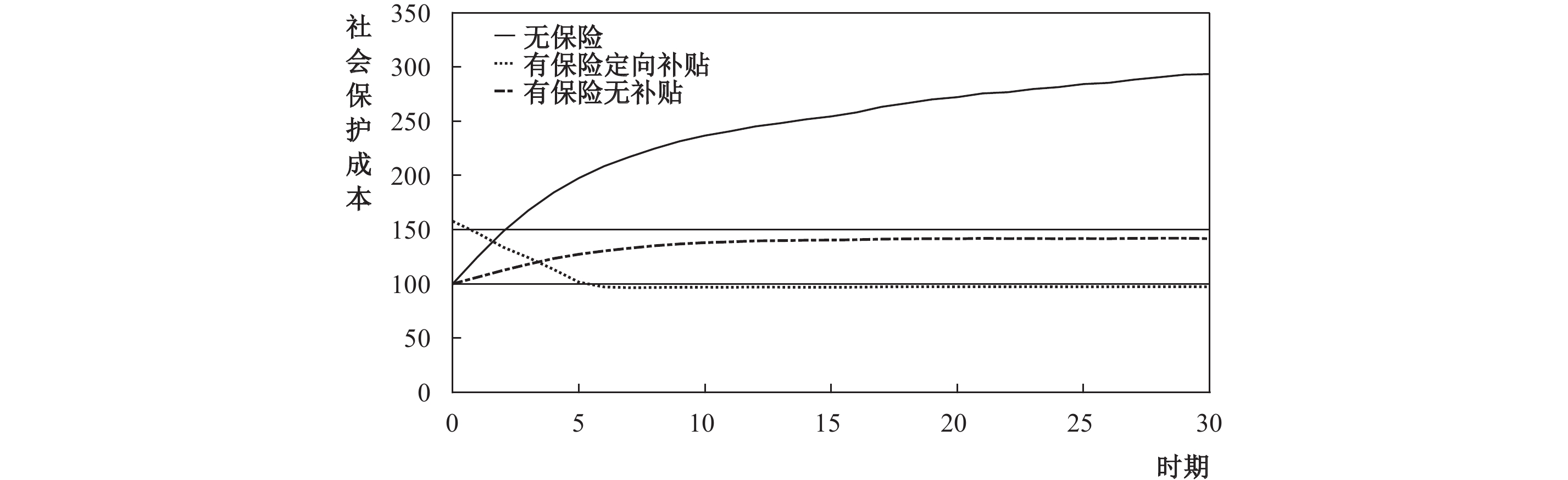图 9 社会保护成本随时间变化情况

① 具体请参考《人类减贫的中国实践》白皮书，国务院新闻办公室，2021。

Micawber阈值最早由Skiba（1978）提出，用以区分个体是否能够进行储蓄和资产良性积累。Zimmerman和Carter（2003）首次将其运用于“贫困陷阱”模型中，用以区分个体是否会陷入“贫困陷阱”。在无保险情形下，资产水平低于Micawber阈值的个体必然会陷入“贫困陷阱”。

③ 由于疾病种类的多样性和治疗费用的差异化，在模型测试过程中，我们加入了随机因素，令疾病冲击和发生概率随机变动（上下变动20%），模型结果依旧稳健，因此本文仅遵循疾病产生的负面冲击和疾病发生概率呈负相关的原则设定了一种可能的分布。

④ 目前，政府对城乡居民基本医疗保险进行了66%左右的保费补贴。本文选取50%的价格补贴进行研究是为了分析在采用略低于现有补贴标准的情况下，医疗保险的长期减贫效果，探讨能否通过降低医疗保险保费补贴来减少长期减贫成本。

⑤ 由式（5）和式（9）可知，均衡状态下个体的流动性成本即为投资的边际收益，投资的边际收益越高，个体的流动性成本也就相应地更高。相较于无保险情形，在有保险无补贴情形下，医疗保险机制的引入会弱化外生疾病冲击的影响，使得投资的边际收益更高，即 ${\lambda }_{I}\left({k}_{t+1}\right)\geqslant {\lambda }_{N}\left({k}_{t+1}\right)$

⑥ 净投资表示家庭在折旧后的上期投资 $\left(1-\delta \right){k}_{t}$ 的基础上，本期额外增加的投资量。净投资也可以理解为在扣除消费 ${c}_{t}$ 和保险费用 $p{I}_{t}$ 后，家庭本期生产收入 $f\left({k}_{t}\right)$ 中用于投资的部分。净投资 ${i}_{t}^{net} > 0$ ，可理解为家庭自愿牺牲部分当期消费，使得未来的生产收入增加，从而加速资产积累。

⑦ 稳健性检验表明，模拟结果对家庭户数（大于100）的选择不敏感，改变家庭户数，模型结果无显著变化。

  陈志钢, 毕洁颖, 吴国宝, 等. 中国扶贫现状与演进以及2020年后的扶贫愿景和战略重点[J]. 中国农村经济, 2019(1): 2–16.  程令国, 张晔. “新农合”: 经济绩效还是健康绩效?[J]. 经济研究, 2012(1): 120–133.  黄晓宁, 李勇. 新农合对农民医疗负担和健康水平影响的实证分析[J]. 农业技术经济, 2016(4): 51–58. DOI:10.13246/j.cnki.jae.2016.04.005  黄征学, 高国力, 滕飞, 等. 中国长期减贫, 路在何方? ——2020年脱贫攻坚完成后的减贫战略前瞻[J]. 中国农村经济, 2019(9): 2–14.  黄薇. 医保政策精准扶贫效果研究——基于URBMI试点评估入户调查数据[J]. 经济研究, 2017(9): 117–132.  景鹏, 郑伟, 贾若, 等. 保险机制能否助推脱贫并守住脱贫成果? ——基于资产积累模型的分析[J]. 经济科学, 2019(2): 104–116.  刘子宁, 郑伟, 贾若, 等. 医疗保险、健康异质性与精准脱贫——基于贫困脆弱性的分析[J]. 金融研究, 2019(5): 56–75.  卢盛峰, 卢洪友. 政府救助能够帮助低收入群体走出贫困吗? ——基于1989—2009年CHNS数据的实证研究[J]. 财经研究, 2013(1): 4–16. DOI:10.16538/j.cnki.jfe.2013.01.001  齐良书. 新型农村合作医疗的减贫、增收和再分配效果研究[J]. 数量经济技术经济研究, 2011(8): 35–52. DOI:10.13653/j.cnki.jqte.2011.08.001  孙武军, 祁晶. 保险保障、家庭资本增长与贫困陷阱[J]. 管理科学学报, 2016(12): 71–82. DOI:10.3969/j.issn.1007-9807.2016.12.007  王翌秋, 刘蕾. 新型农村合作医疗保险、健康人力资本对农村居民劳动参与的影响[J]. 中国农村经济, 2016(11): 68–81.  解垩. 医疗保险与城乡反贫困: 1989—2006[J]. 财经研究, 2008(12): 68–83. DOI:10.3969/j.issn.1001-9952.2008.12.006  张楠, 张栋浩, 李建军, 等. 长期减贫的未雨绸缪——来自扶贫改革试验区的证据[J]. 财贸经济, 2020(3): 20–35. DOI:10.3969/j.issn.1002-8102.2020.03.003  赵思诚, 杨青, 许庆. 社会保障、信贷获得与农业生产——来自新型农村合作医疗制度的证据[J]. 财经研究, 2019(11): 45–56. DOI:10.16538/j.cnki.jfe.2019.11.004  郑晓冬, 上官霜月, 陈典, 等. 有条件现金转移支付与农村长期减贫: 国际经验与中国实践[J]. 中国农村经济, 2020(9): 124–144.  Akotey J O, Adjasi C. The impact of microinsurance on household asset accumulation in Ghana: An asset index approach[J]. The Geneva Papers on Risk and Insurance-Issues and Practice, 2014, 39(2): 304–321. DOI:10.1057/gpp.2014.6  Barrett C B, Carter M R. The economics of poverty traps and persistent poverty: Empirical and policy implications[J]. The Journal of Development Studies, 2013, 49(7): 976–990. DOI:10.1080/00220388.2013.785527  De Nicola F. The impact of weather insurance on consumption, investment, and welfare[J]. Quantitative Economics, 2015, 6(3): 637–661. DOI:10.3982/QE300  Foster J, Greer J, Thorbecke E. A class of decomposable poverty measures[J]. Econometrica, 1984, 52(3): 761–766. DOI:10.2307/1913475  Ikegami M, Carter M R, Barrett C B, et al. Poverty traps and the social protection paradox[M]. London: The University of Chicago Press, 2019.  Janzen S A, Carter M R, Ikegami M. Can insurance alter poverty dynamics and reduce the cost of social protection in developing countries?[J]. Journal of Risk and Insurance, 2021, 88(2): 293–324. DOI:10.1111/jori.12322  McPeak J. Contrasting income shocks with asset shocks: Livestock sales in Northern Kenya[J]. Oxford Economic Papers, 2004, 56(2): 263–284. DOI:10.1093/oep/gpf040  Skiba A K. Optimal growth with a convex-concave production function[J]. Econometrica, 1978, 46(3): 527–539. DOI:10.2307/1914229  Zimmerman F J, Carter M R. Asset smoothing, consumption smoothing and the reproduction of inequality under risk and subsistence constraints[J]. Journal of Development Economics, 2003, 71(2): 233–260. DOI:10.1016/S0304-3878(03)00028-2